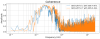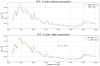Reports 1-1 of 1
VIS (SR2)
fabian.arellano - 15:39 Wednesday 28 September 2022 (22229) Print this report
Comment to Inter-calibration between LVDT, geophone, and IXV seismometer. (20873)

Using the data measured yesterday (klog 22217), I calculated the calibration factor between the output of seismometer on the ground (2nd floor) SEIS and the term (GEO-LVDT).

Per klog 20873, the aim is to calculate a such that a * SEIS = GEO-LVDT, in a frequency range where there is high coherence (Fig. 1). The value of a was calculated by minimizing the RMS value of the function  (GEO-LVDT) - a * SEIS.

The results are as follows:

• Frequency range: from 160 to 500 mHz.
• IP-L, Y Arm: a = 1.21104 (Fig. 2).
• IP-T, X Arm: a =  1.17220 (Fig. 3).

These calibration factors still need to be written in the appropriate filter banks, and then the performance of the sensor correction can be assessed.

Images attached to this comment×

×